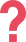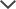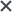## What is the STAR Math Test?

The  STAR Assessments are a series of short tests administered by Renaissance Learning to children in grades K-12. The STAR Math is a multiple-choice test used to measure math achievement and progression and provide teachers and parents with actionable insights into each student's math proficiency.

Since the test provides an exact picture of students' abilities, it predicts performance in standardized state testing. Therefore, it could be used as an indicator for selection into Accelerated Math programs.

## STAR Math Test Format & Administration

The STAR Math test is a computer-adaptive test that provides a personalized assessment by adapting to each student's learning level. Each question's difficulty is determined by how the student has answered the previous question.

The STAR Math Enterprise version is designated for screening and benchmarking periodic assessment, consists of 34 questions, and is administered a few times a year. The test also has a shortened version set for progress monitoring that consists of 24 questions and is designed to be distributed more often.

The test is not timed, and students may take as much time as they need to complete it, although some questions do have a time limit to keep the test moving and for security reasons. On average, the STAR Math test takes approximately 20 minutes to complete.

Students are only allowed to use pen and paper during the test and cannot use external resources such as calculators. However, in questions that require complex calculations or formulas, a calculator or other assisting tools may appear on the examinee's test screen.

## Renaissance Math Domains & STAR Sample Questions

The STAR Math focuses on measuring student performance and skills aligned with the US Common Core State Standards for Mathematics. All questions on the test are multiple-choice questions with four answer choices and cover the following four domains

• Numbers and Operations – Covers essential arithmetic operations (addition, subtraction, multiplication, and division) in solving mathematical problems.
 STAR Sample Question #1: Numbers and Operations Evaluate 4 + 5(8 ÷ 2) - 7.   15 17 27 29 Answer & Explanation ▼ | ▲ The correct answer is B. PEMDAS is the order of operations and stands for: Parentheses; Exponents; Multiplication; Division; Addition; Subtraction Instead of calculating left to right, you must work in the order PEMDAS states. Multiplication and division have the same priority, so you must calculate those from left to right. The same goes for addition and subtraction. In 4 + 5(8 ÷ 2) - 7, you must calculate inside the parentheses first; 8 ÷ 2 = 4, so: 4 + 5(4) - 7 Next, multiply across the parentheses; 5 × 4 = 20, so: 4 + 20 – 7 Now, you can operate left to right as there is only addition and subtraction left: 24 – 7 = 17. Therefore, the correct answer is B.

 STAR Sample Question #2: Numbers and Operations Which of the following expressions is equivalent to (6 × 9) × 2 + 6 ? (6+6)x2+9 6+2x(9+6) (9x2)x6+6 (2x6)x6+9 Answer & Explanation ▼ | ▲ The correct answer is (C). When multiplying, you can change the order of the numbers and get the same result. In addition, you can also move the parentheses and still get the same result. Look at the original expression: (6 × 9) × 2 + 6 → 6 × (9 × 2) + 6 → (9 × 2) × 6 + 6 In this expression, the parentheses were moved from 6 × 9 to 9 × 2. Then, the places of 9 × 2 and × 6 were switched. Note that + 6 did not change its place. Thus, this expression is equivalent to the expression in answer choice (C). Therefore, the correct answer is (C).

• Algebra - Covers the applications of algebraic concepts, such as basic arithmetic skills, solving equations and inequalities, identification of points on the coordinate plane, expression simplification, etc.
 STAR Sample Question #3: Algebra Solve 6x – 5 = 10 + 3x.   5/3 3 9/5 5 Answer & Explanation ▼ | ▲ The correct answer is D. When solving equations, it is essential that whichever operation you perform, you must apply it to both sides of the equal's sign—for example, adding three to both sides. To solve this open sentence, make sure all the letters are on the same side. Move the smaller amount, which is the 3x. To remove it from the right-hand side, subtract 3x and then do the same to the left-hand side, so the equation is still balanced: 6x – 3x – 5 = 10 + 3x – 3x Now simplify the letters on each side: 3x – 5 = 10 Next, make sure the numbers are on the other side. Move the numbers to the right-hand side as the letter is now on the left-hand side. To remove -5 from the left, you must add 5, so they cancel to zero. Do the same to the right-hand side also: 3x – 5 + 5 = 10 + 5 Simplify the numbers on both sides: 3x = 15 Finally, divide both sides by 3 to get x isolated: x = 5 Therefore, the correct answer is D.
 STAR Sample Question #4: Algebra Solve.5 13 14 15 Answer & Explanation ▼ | ▲ The correct answer is (D). To find what number goes in the box, you need to understand how subtraction works. If subtracting a number from 19 gives as a result four, then subtracting four from 19 must give that same number. Simply put, if: 19 – = 4 Then, 19 – 4 = Therefore, you need to subtract four from 19. 19 – 4 = 15, and that is answer (D).
• Geometry and Measurement – Covers the understanding of geometric concepts, including identifying and classifying figures, line segments, angles, etc., using measurement units, conversion, and calculation of geometric measures such as circumference, area, surface area, and volume.
 STAR Sample Question #5: Geometry and Measurement What is the area of the following figure?34 44 84 105 Answer & Explanation ▼ | ▲ The correct answer is C. The formula for the area of a parallelogram is b x h, where b is the flat base of the parallelogram and h is the vertical height. The base and the height must be perpendicular to each other. The two labeled sides that are perpendicular are seven and 12. The vertical height, 12, can be drawn inside or outside the shape. The important thing is that if you were to move it sideways, it would meet the side of seven at 90 degrees. So, to find the area: Area = b x h = 7 x 12 = 84 square units. Therefore, the correct answer is C.
 STAR Sample Question #6: Geometry and Measurement Which of the following triangles is an isosceles triangle? A)B)C)D)Answer & Explanation ▼ | ▲ The correct answer is (B).An isosceles triangle is a triangle with two equal angles. Check the answer choices:Answer (A): There are three different angles.Answer (B): There are two equal angles (72°).Answer (C): There are three different angles.Answer (D): There are three different angles.Answer choice (B) is the only triangle with two equal angles.Therefore, answer (B) is the correct answer.
• Data Analysis, Statistics, and Probability – Covers methods of organizing, reading, interpreting, and analyzing data representations such as charts and graphs and the ability to use probability concepts to make predictions.
 STAR Sample Question #7: Data Analysis, Statistics, and Probability A six-sided die is rolled as part of an experiment. If the die sides are numbered from one to six, what are the odds that it will fall below 5?   1/2 1/3 4/6 1 Answer & Explanation ▼ | ▲ The correct answer is C. To calculate the odds of an event, you can divide the number of favorable outcomes by the number of overall outcomes. There are six outcomes overall based on the numbers from one to six. You need to calculate the odds of the outcome being below five. Four possible outcomes are below five (the numbers one, two, three, and four). Therefore, the odds of that happening are 4/6.
 STAR Sample Question #8: Data Analysis, Statistics, and Probability Use the line plot below to answer the question.How many dogs were at least 50 kilograms in weight? 3 7 9 10 Answer & Explanation ▼ | ▲ The correct answer is (C). Each cross on this line plot represents one dog. Thus, there were three dogs who weighed 50 kg. The question asks how many weighed at least 50 kg. At least 50 kg means 50 kg or more, so 50 kg or 60 kg. You must count the number of dogs who weighed 60 kg and add them on to the three dogs who weighed 50 kg. There are six dogs who weighed 60 kg. Therefore, the number of dogs who weighed at least 50 kg were: 3 + 6 = 9. If you answered (A), you just counted the dogs who weighed exactly 50 kg. If you answered (B), you counted the dogs who weighed less than 50 kg. At least means the same as or more. If you answered (D), you counted the dogs who weighed at most 50 kg.

## STAR Math Scores

The STAR Math Score Report (or Parent Report) summarizes the student's performance on the test. This report contains norm-referenced scores, comparing the student's results to those of other students who have taken the test. There are four different score measures:

• Scaled Score (SS): This scoring method provides a single common scale for measuring students' math achievement from first through twelfth grade. The Scaled Scores are formed from the conversion of all the STAR Math test scores into a standard scale, ranging from 0 to 1400, estimating the location of each student on a math ability scale. Scaled scores are therefore valid as a way of monitoring performance over time and across grades.
• Grade Equivalent (GE): This score indicates the student's rank in the grade placement level for which their score is typical (regardless of the student's actual grade). Grade Equivalent STAR Math scores range from 0.0 to 12.9+, indicating grade placement and months of the school year. For example, a GE of 7.5 means that the student scored as well as did the typical student in the middle of seventh grade (the fifth month of the school year, to be exact).
• Percentile Rank (PR): This scoring system measures students' performance relative to other students in the same grade and indicates the percentage of students at the same point of time in the school year who obtained a lower score than the individual student. For example, a Percentile Rank of 70 states that the student performed better than 70% of students in that grade at the same time of the year. Percentile Ranks range from 1 to 99.
TestPrep-Online's complete STAR Preparation Packs include
Tutorials, Practice Tests, and Expert Tips from \$59

## How to prepare for the STAR Math Test?

The Renaissance Star Math Assessment can significantly impact a child's academic career. Moreover, since STAR tests primarily examine students' math proficiency according to the school curriculum, practicing for the exam can also enhance the students' ability and knowledge and help them excel in school in a regular studying routine.

The test may be somewhat intimidating for some students since math anxiety affects many people and start appearing as early as first grade. However, preparing before the exam and working on the student's motivation can significantly reduce the pressure accompanying the STAR Math Test. Here are some helpful tips to help prepare for test day:

• Refresh your familiarity with mathematical definitions, such as decompose, regrouping, numerator and denominator, quadrilateral, mean, mode, median, etc.; It is highly likely to encounter some of these (and other) terms in the STAR Math, so it is crucial to make sure you go through standard terms that you are not comfortable with.
• Practice reading and interpreting graphs, pictographs, dot plots, and comparing tables and graphs. Graph problems are common in STAR tests, and therefore it is essential to make sure you are familiar with the different ways of data presentation and analysis.
• Make sure you know and understand different units of measurement. Some questions may require converting from standard units (inches, pounds, pints, yards) to metric units (centimeters, liters, grams). Practice these conversions.

The optimal preparation technique is to practice answering the same types of questions that are asked on the actual STAR Math test. Practicing the different mathematical topics and various question types enables students to deepen their understanding of the material and enhance their problem-solving skills. Our STAR Math Practice Packs are tailored for this purpose precisely, simulating the real test conditions and including multiple sample questions and complete practice tests.

## Online STAR Math Practice Test

TestPrep-Online provides extensive STAR Math preparation packs designated to upgrade students' test performance and help them improve their mathematical skills with ease.

Our packs include full-length practice tests, accurately simulating the real STAR exam, alongside additional practice quizzes with many sample questions that allow practicing as much as needed. All practice questions are coupled with complete solutions and thorough explanations, ensuring everything is well understood.

The practice pack also contains a complete and comprehensive STAR Math study guide with an elaborated manual on the various mathematical concepts of the STAR, together with insightful solving tips and preparation methods. All practice tests on our packs are administered in a user-friendly online system and include elaborated score reports to monitor progress over time.

## FAQ

How many questions are on the STAR Math test?

The STAR Math Enterprise version consists of 34 questions, and the STAR Math for progress monitoring consists of 24 questions.

Is the STAR Math test timed?

Generally, the STAR Math test is not timed, and students may take as much time as they need to complete. However, some questions may have a three-minute time limit to keep the test flowing and for security reasons.

How long does the STAR Math test take?

On average, the STAR Math test takes approximately 20 minutes to complete. However, some students may need more time.

What is a good score on the STAR Math test?

The STAR Math Percentile Rank (PR) can indicate how well a student has performed relative to other students in the same grade. Click here for STAR Math scaled scores and Percentile Rank by the grade-level chart.

Can you use a calculator on the STAR Math test?

Any external resources, such as calculators, are strictly prohibited. However, some algebra and geometry questions will present an on-screen calculator and formula reference sheet that can be used to answer them.

Need HelpNeed HelpPlease fill out the form below and we will contact you soon.
Your message was sent. We will contact you shortly.
There was a problem sending your message. Please try again in a few minutes.